Note: This is a working paper which will be expanded/updated frequently. All suggestions for improvement are welcome. The directory deleeuwpdx.net/pubfolders/splines has a pdf version, the complete Rmd file with all code chunks, the bib file, and the R and C source code.

# 1 Introduction

To define spline functions we first define a finite sequence of knots $$T=\{t_j\}$$, with $$t_1\leq\cdots\leq t_p,$$ and an order $$m$$. In addition each knot $$t_j$$ has a multiplicity $$m_j$$, the number of knots equal to $$t_j$$. We suppose throughout that $$m_j\leq m$$ for all $$j$$.

A function $$f$$ is a spline function of order $$m$$ for a knot sequence $$\{t_j\}$$ if

1. $$f$$ is a polynomial $$\pi_j$$ of degree at most $$m-1$$ on each half-open interval $$I_j=[t_j,t_{j+1})$$ for $$j=1,\cdots,p$$,
2. the polynomial pieces are joined in such a way that $$\mathcal{D}^{(s)}_-f(t_j)=\mathcal{D}^{(s)}_+f(t_j)$$ for $$s=0,1,\cdots,m-m_j-1$$ and $$j=1,2,\cdots,p$$.

Here we use $$\mathcal{D}^{(s)}_-$$ and $$\mathcal{D}^{(s)}_+$$ for the left and right $$s^{th}$$-derivative operator. If $$m_j=m$$ for some $$j$$, then requirement 2 is empty, if $$m_j=m-1$$ then requirement 2 means $$\pi_j(t_j)=\pi_{j+1}(t_j)$$, i.e. we require continuity of $$f$$ at $$t_j$$. If $$1\leq m_j<m-1$$ then $$f$$ must be $$m-m_j-1$$ times differentiable, and thus continuously differentiable, at $$t_j$$.

In the case of simple knots (with multiplicity one) a spline function of order one is a step function which steps from one level to the next at each knot. A spline of order two is piecewise linear, with the pieces joined at the knots so that the spline function is continuous. Order three means a piecewise quadratic function which is continuously differentiable at the knots. And so on.

# 2 Basic Splines

Alternatively, a spline function of order $$m$$ can be defined as a linear combination of B-splines (or basic splines) of order $$m$$ on the same knot sequence. A $$B$$-spline of order $$m$$ is a spline function consisting of at most $$m$$ non-zero polynomial pieces. A $$B$$-spline $$\mathcal{B}_{j,m}$$ is determined by the $$m+1$$ knots $$t_j\leq\cdots\leq t_{j+m}$$, is zero outside the interval $$[t_j,t_{j+m})$$, and positive in the interior of that interval. Thus if $$t_j=t_{j+m}$$ then $$\mathcal{B}_{j,m}$$ is identically zero.

For an arbitrary finite knot sequence $$t_1,\cdots,t_p$$, there are $$p-m$$ $$B$$-splines to of order $$m$$ to be considered, although some may be identically zero. Each of the splines covers at most $$m$$ consecutive intervals, and at most $$m-1$$ different $$B$$-splines are non-zero at each point.

## 2.1 Boundaries

$$B$$-splines are most naturally and simply defined for doubly infinite sequences of knots, that go to $$\pm\infty$$ in both directions. In that case we do not have to worry about boundary effects, and each subsequence of $$m+1$$ knots defines a $$B$$-spline. For splines on finite sequences of $$p$$ knots we have to decide what happens at the boundary points.

There are $$B$$-splines for $$t_j,\cdots,t_{j+m}$$ for all $$j=1,\cdots,p-m$$. This means that the first $$m-1$$ and the last $$m-1$$ intervals have fewer than $$m$$ splines defined on them. They are not part of what De Boor (2001), page 94, calls the basic interval. For doubly infinite sequences of knots there is not need to consider such a basic interval.

If we had $$m$$ additional knots on both sides of our knot sequence we would also have $$m$$ additional $$B$$-splines for $$j=1-m,\cdots,0$$ and $$m$$ additional $$B$$-splines for $$j=p-m+1,\cdots,p$$. By adding these additional knots we make sure each interval $$[t_j,t_{j+1})$$ for $$j=1,\cdots,p-1$$ has $$m$$ $$B$$-splines associated with it. There is stil some ambiguity on what to do at $$t_p$$, but we can decide to set the value of the spline there equal to the limit from the left, thus making the $$B$$-spline left-continuous there.

In our software we will use the convention to define our splines on a closed interval $$[a,b]$$ with $$r$$ interior knots $$a<t_1<\cdots<t_r<b$$, where interior knot $$t_j$$ has multiplicity $$m_j$$. We extend this to a series of $$p=M+2m$$ knots, with $$M=\sum_{j=1}^r m_j$$, by starting with $$m$$ copies of $$a$$, appending $$m_j$$ copies of $$t_j$$ for each $$j=1,\cdots,r$$, and finishing with $$m$$ copies of $$b$$. Thus $$a$$ and $$b$$ are both knots with multiplicity $$m$$. This defines the extended partition (Schumaker (2007), p 116), which is just handled as any knot sequence would normally be.

## 2.2 Normalization

$$B$$-splines can be defined in various ways, using piecewise polynomials, divided differences, or recursion. The recursive definition, first used as a defining condition by De Boor and Höllig (1985), is the most convenient one for computational purposes, and that is the one we use.

But first, the conditions we have mentioned only determine the $$B$$-spline up to a normalization. There are two popular ways of normalizing $$B$$-splines. The $$N$$-splines $$N_{j,m}$$, a.k.a. the normalized $$B$$-splines $$j$$ or order $$m$$, satisfies $\begin{equation}\label{E:nsum} \sum_{j}N_{j,m}(t)=1. \end{equation}$ Note that in general this is not true for all $$t$$, but only for all $$t$$ in the basic interval.

Alternatively we can normalize to $$M$$-splines, for which $\begin{equation}\label{E:mint} \int_{-\infty}^{+\infty}M_{j,m}(t)dt=\int_{t_j}^{t_{j+k}}M_{j,m}(t)dt=1. \end{equation}$ There is the simple relationship $\begin{equation}\label{E:NM} N_{j,m}(t)=\frac{t_{j+m}-t_j}{m}\ M_{j,m}(t). \end{equation}$

## 2.3 Recursion

The recursive definition, due independently to Cox (1972) for simple knots and to De Boor (1972) in the general case, is $\begin{equation}\label{E:Mspline} M_{j,m}(t)=\frac{t-t_j}{t_{j+m}-t_j}M_{j,m-1}(t)+\frac{t_{j+m}-t}{t_{j+m}-t_j}M_{j+1,m-1}(t), \end{equation}$ or $\begin{equation}\label{E:Nspline} N_{j,m}(t)=\frac{t-t_j}{t_{m+j-1}-t_j}N_{j,m-1}(t)+\frac{t_{j+m}-t}{t_{j+m}-t_{j+1}}N_{j+1,m-1}(t). \end{equation}$

A basic result in the theory of $$B$$-splines is that the different $$B$$-splines are linearly independent and form a basis for the linear space of spline functions (of a given order and knot sequence).

# 3 Computations

Before introducing our new C code we review some the approaches we have used in the past. This will also give us the opportunity to make some comparisons.

## 3.1 Low Order Splines

The R code in lowSpline.R has three functions to compute splines of order one, two, and three. It does not acknowledge any boundary values, so only looks at $$B$$-splines on an interval spanned by interior knots. The formulas we use are

$N_{j,1}(x)=\begin{cases}1&\text{ if }t_j\leq x<t_{j+1},\\0&\text{ otherwise}\end{cases}.$

$N_{j,2}(x)=\begin{cases}\frac{x-t_j}{t_{j+1}-t_j}&\text{ if }t_j\leq x<t_{j+1},\\\frac{t_{j+2}-x}{t_{j+2}-t_{j+1}}&\text{ if }t_{j+1}\leq x<t_{j+2},\\0&\text{ otherwise}\end{cases}.$

$N_{j,3}(x)=\begin{cases}\frac{(x-t_j)^2}{(t_{j+1}-t_j)(t_{j+2}-t_j)}&\text{ if }t_j\leq x<t_{j+1},\\ \frac{(x-t_j)(t_{j+2}-x)}{(t_{j+2}-t_j)(t_{j+2}-t_{j+1})}+\frac{(x-t_{j+1})(t_{j+3}-x)}{(t_{j+3}-t_{j+1})(t_{j+2}-t_{j+1})}&\text{ if }t_{j+1}\leq x<t_{j+2},\\ \frac{(t_{j+3}-x)^2}{(t_{j+3}-t_{j+1})(t_{j+3}-t_{j+2})}&\text{ if }t_{j+2}\leq x<t_{j+3},\\ 0&\text{ otherwise}\end{cases}.$

In the example of Ramsay (1988) the knots are 0.0, 0.3, 0.5, 0.6, and 1.0 (in the example 0 and 1 are endpoints of the interval, but we’ll just treat them as interior knots in a longer sequence). Also note that Ramsay computes $$M$$-splines, while we compute $$N$$-splines.

We start with the $$p-m=5-2=3$$ $$B$$-splines of order 2. The basic interval is $$[0.3,0.6]$$.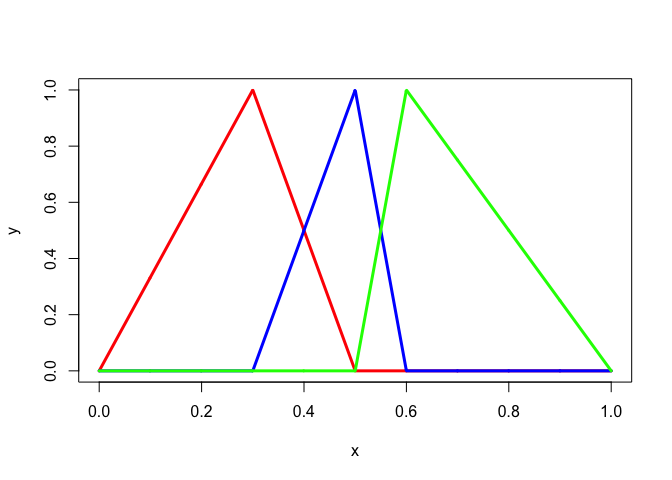Figure 1: Piecewise Linear Splines with Simple Knots

There are only two $$B$$-splines of order 3 on these knots, and the basic interval is empty.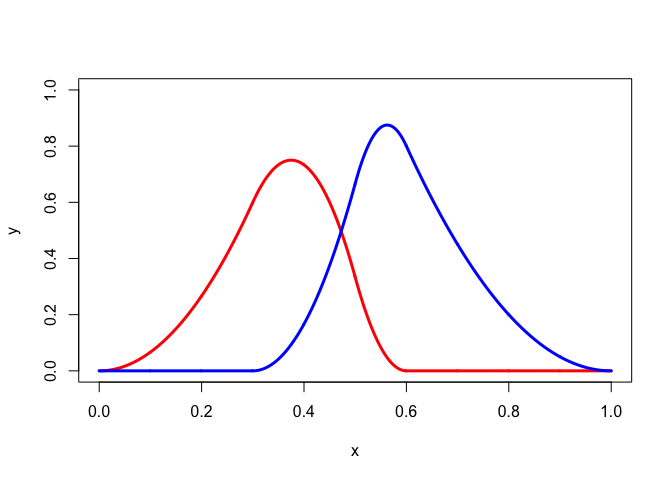Figure 2: Piecewise Quadratic Splines with Simple Knots

The programs also work for multiple knots. Consider the example from De Boor (2001), page 92. The knots are 0, 1, 1, 3, 4, 6, 6, 6, and the order is three. The basic interval is $$[1,6]$$.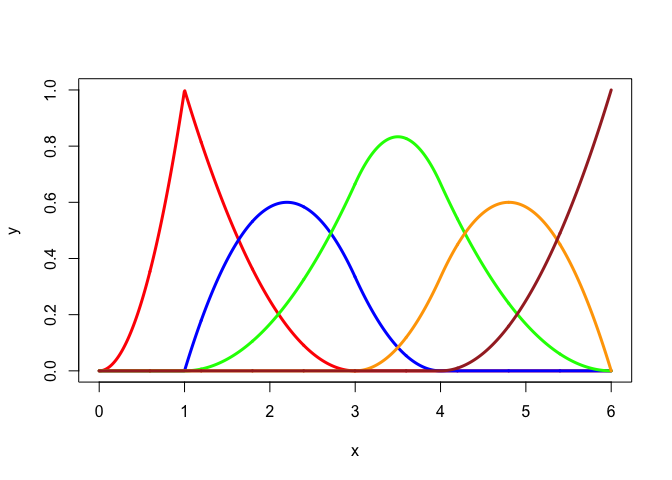Figure 3: Piecewise Quadratic Splines with Multiple Knots

In De Boor (2001), p 89, we find another example in which there are only two distinct knots, an infinite sequence of zeroes, followed by an infinite sequence of ones. In this case there are only $$m$$ different $$B$$-splines, restriction to $$[0,1]$$ of the familiar polynomials $B_{j,m-1}(x)=\binom{m-1}{j}x^{m-1-j}(1-x)^{j}$ for $$j=0,\cdots,m-1$$.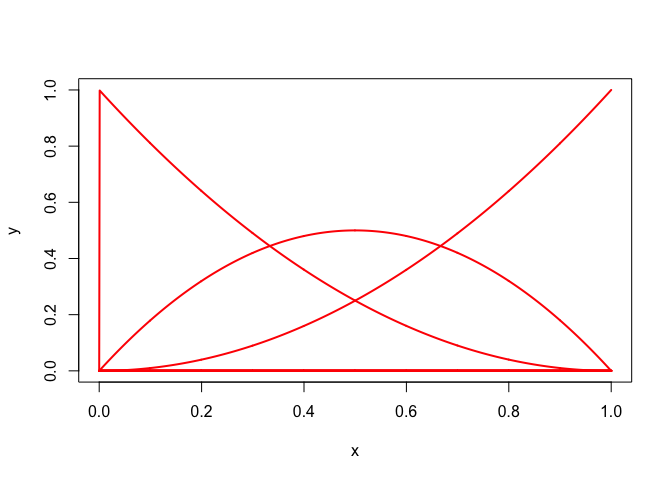## 3.2 Using GSL

The GNU Scientific Library (Galassi et al. (2016)) has $$B$$-spline code. The function gslSpline() in R calls the compiled gslSpline.c, which is linked with the relevant code from libgsl.dylib. We use the Ramsay example again. The GSL implementation automatically adds the extra boundary knots for the extended partition, which makes the basic interval $$[0,1]$$.

knots <- c(0,.3,.5,.6,1)
order <- 3
x<-seq(0,1,length = 1001)
h <- matrix (0, 1001, 6)
for (i in 1:1001)
h[i,] <- gslSpline (x[i], order, knots)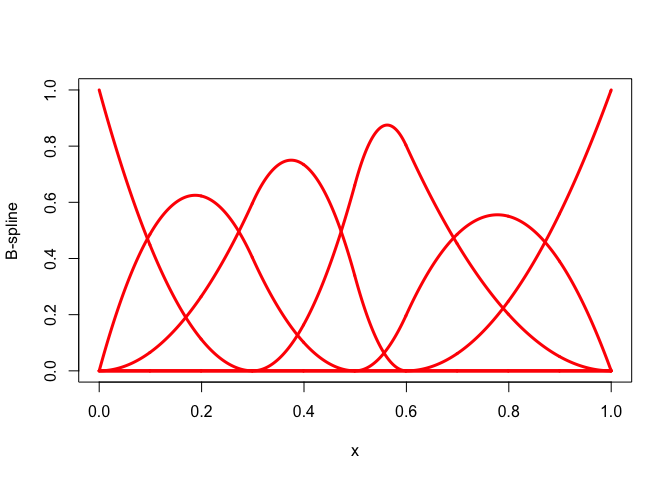Figure 5: Piecewise Quadratic Splines using GSL

## 3.3 Using Recursion

We have previously published spline function code, using an R interface to C code, in J De Leeuw (2015). That code translated the Fortran code in an unpublished note by Sinha (n.d.) to C. There are some limitations associated with this implementation. First, it is limited to extended partitions with simple inner knots. Second, the function to compute $$B$$-spline values recursively calls itself, using the basic relation $$\eqref{E:Nspline}$$, which is computationally not necessarily the best way to go.

innerknots <- c( .3, .5, .6)
degree <- 2
lowknot <- 0
highknot <- 1
x<-seq(0,1,length = 1001)
h <- sinhaBasis (x, degree, innerknots, lowknot, highknot)Figure 6: Piecewise Quadratic Splinesusing Recursion

## 3.4 De Boor

In this paper we implement the basic BSPLVB algorithm from De Boor (2001), page 111, for normalized $$B$$-splines. There are two auxilary routines, one to create the extended partition, and one that uses bisection to locate the knot interval in which a particular value is located (Schumaker (2007), p 191). The function bsplineBasis() takes an arbitrary knot sequence. It can be combined with extendPartition(), which uses inner knots and boundary points to create the extended partion.

## 3.5 Illustrations

For our example, which is the same as the one from figure 1 in Ramsay (1988), we choose $$a=0$$, $$b=1$$, with simple interior knots 0.3, 0.5, 0.6. First the step functions, which have order 1.
innerknots <- c(.3,.5,.6)
multiplicities <- c(1,1,1)
order <- 1
lowend <- 0
highend <- 1
x <- seq (1e-6, 1-1e-6, length = 1000)
knots <- extendPartition (innerknots, multiplicities, order, lowend, highend)\$knots
h <- bsplineBasis (x, knots, order)
k <- ncol (h)
par (mfrow=c(2,2))
for (j in 1:k) {
ylab <- paste("B-spline", formatC(j, digits = 1, width = 2, format = "d"))
plot (x, h[, j], type="l", col = "RED", lwd = 3, ylab = ylab, ylim = c(0,1))
}